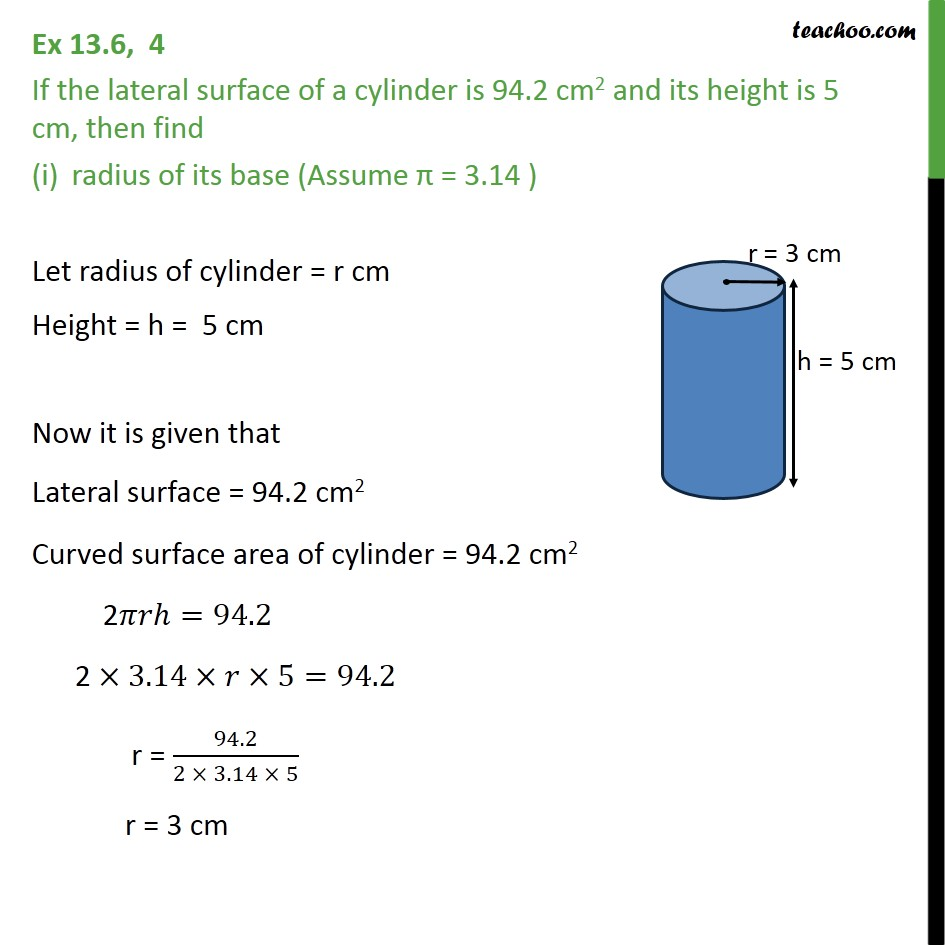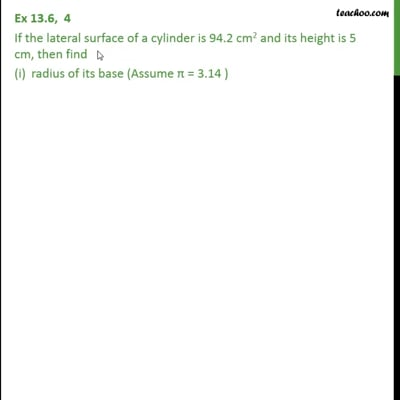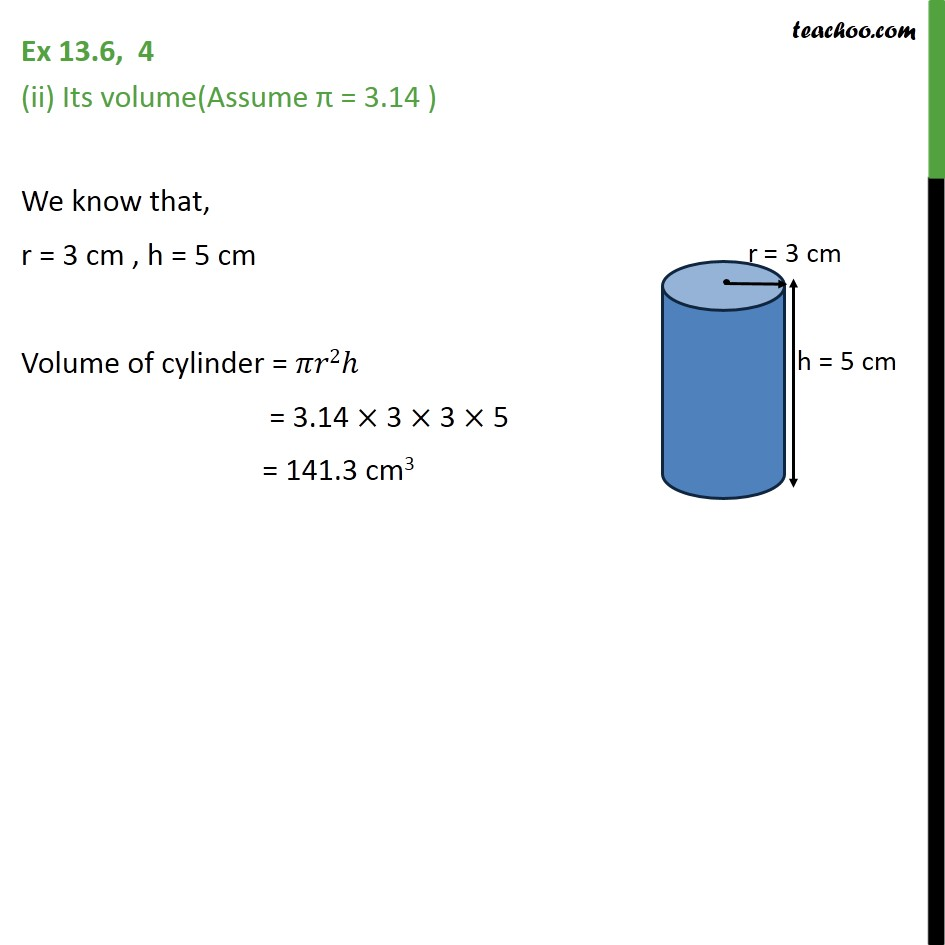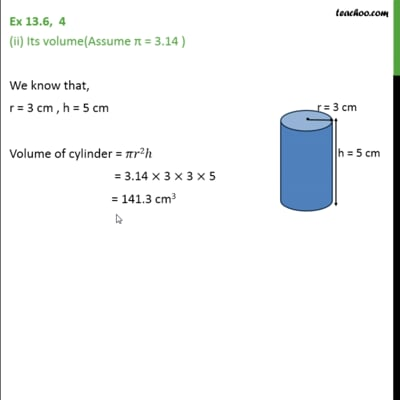Volume Of Cylinder

Chapter 13 Class 9 Surface Areas and Volumes
Concept wiseThis video is only available for Teachoo black usersThis video is only available for Teachoo black users

Introducing your new favourite teacher - Teachoo Black, at only ₹83 per month

### Transcript

Ex 13.6, 4 If the lateral surface of a cylinder is 94.2 cm2 and its height is 5 cm, then find radius of its base (Assume π = 3.14 ) Let radius of cylinder = r cm Height = h = 5 cm Now it is given that Lateral surface = 94.2 cm2 Curved surface area of cylinder = 94.2 cm2 2𝜋𝑟ℎ=94.2 2 ×3.14×𝑟×5=94.2 r = 94.2/(2 × 3.14 × 5) r = 3 cm Ex 13.6, 4 (ii) Its volume(Assume π = 3.14 ) We know that, r = 3 cm , h = 5 cm Volume of cylinder = 𝜋𝑟2ℎ = 3.14 × 3 × 3 × 5 = 141.3 cm3